Courses

# Partial Derivatives And Euler's Equation NAT

## 10 Questions MCQ Test Topic wise Tests for IIT JAM Physics | Partial Derivatives And Euler's Equation NAT

Description
This mock test of Partial Derivatives And Euler's Equation NAT for Physics helps you for every Physics entrance exam. This contains 10 Multiple Choice Questions for Physics Partial Derivatives And Euler's Equation NAT (mcq) to study with solutions a complete question bank. The solved questions answers in this Partial Derivatives And Euler's Equation NAT quiz give you a good mix of easy questions and tough questions. Physics students definitely take this Partial Derivatives And Euler's Equation NAT exercise for a better result in the exam. You can find other Partial Derivatives And Euler's Equation NAT extra questions, long questions & short questions for Physics on EduRev as well by searching above.
*Answer can only contain numeric values
QUESTION: 1

###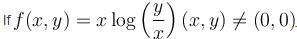then the value of x2fxx + 2xyfxy + y2fyy =

Solution: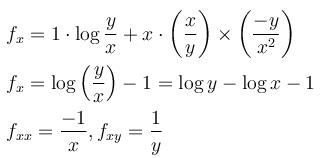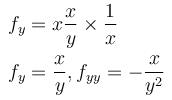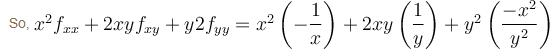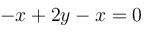*Answer can only contain numeric values
QUESTION: 2

### If u(x, y) = eαx+βy satisfy the condition uxx - 7uxy + 12uyy = 0. α2 - 7αβ + β2 = ____

Solution:

We are given with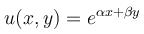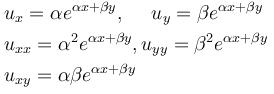put these values in the given relation, we get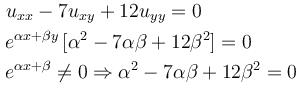*Answer can only contain numeric values
QUESTION: 3

### Let f = yx, what is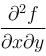at x = 2, y = 1

Solution:

f = yx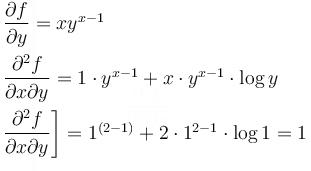*Answer can only contain numeric values
QUESTION: 4

If  z = 2(ax + by)2 – (x2 + y2)  and  a2 + b2 = 1 then find the value of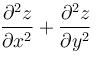Solution: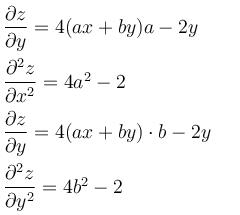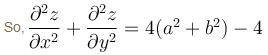= 4(1) – 4 = 0

*Answer can only contain numeric values
QUESTION: 5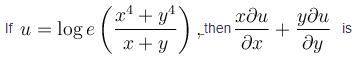Solution: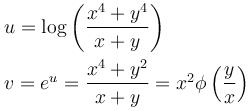v is homogeneous function of degree 3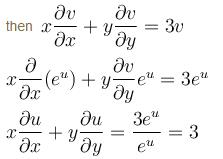*Answer can only contain numeric values
QUESTION: 6

If u = log(tan x + tan y + tan z), then (sin 2x) ux + (sin 2y)uy + sin 2z)uz is equal to

Solution: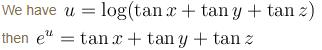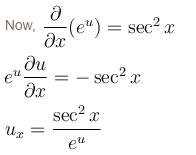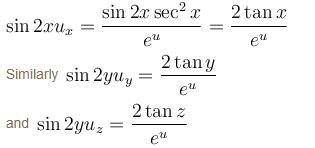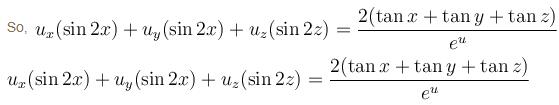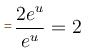*Answer can only contain numeric values
QUESTION: 7

If  f(x, y) = x3y – xy3  then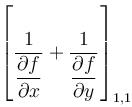Solution: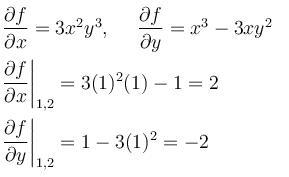1 – 12 = –11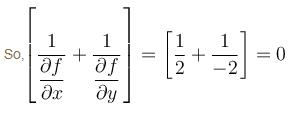*Answer can only contain numeric values
QUESTION: 8

If w = x2cos xy, then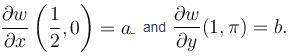Find the value of a + b.

Solution:

We have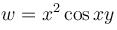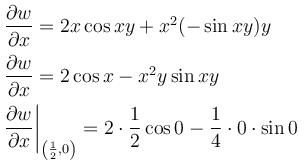= 1 - 0 = 1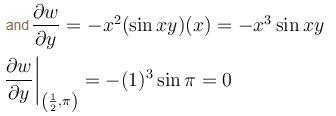a + b = 1

*Answer can only contain numeric values
QUESTION: 9

If v = log(x2 + y2) then vxx + vyy equal to

Solution: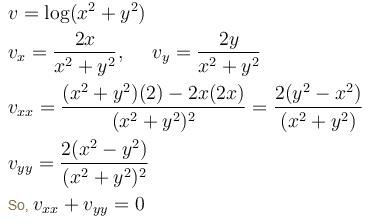*Answer can only contain numeric values
QUESTION: 10

If  z = eax+by f(ax - by) then the value of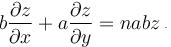The value of n is

Solution: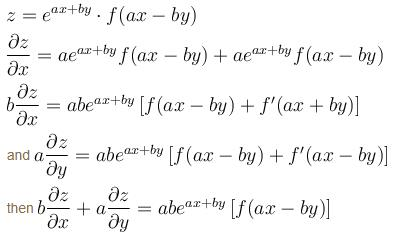= 2abz